So-Bogus A c++ sparse block matrix library aimed at Second Order cone problems
bogus::MecheFrictionProblem Class Reference

struct  Options

## Public Types

enum  Algorithm {
GaussSeidel = 0, ProjectedGradient = 1, MatrixFreeGaussSeidel, ADMM,
DualAMA
}

## Public Member Functions

void fromPrimal (unsigned int NObj, const unsigned int *ndof, const double *const *MassMat, const double *f_in, unsigned int n_in, const double *mu_in, const double *E_in, const double *w_in, const int *const ObjA, const int *const ObjB, const double *const HA[], const double *const HB[])
Allocates and sets up the primal friction problem. More...

double solve (double *r, double *v, bool staticProblem, double problemRegularization, const Options &options)
Solves the friction problem. More...

double solve (double *r, double *v, int maxThreads=0, double tol=0., unsigned maxIters=0, bool staticProblem=false, double regularization=0., bool useInfinityNorm=false, bool useProjectedGradient=false, unsigned cadouxIters=0)
Solves the friction problem (old interface) More...

void computeDual (double regularization)
Computes the dual from the primal.

void reset ()
Cleams up the problem, then allocates a new PrimalFrictionProblem and make m_primal point to it.

unsigned nDegreesOfFreedom () const

unsigned nContacts () const

void setOutStream (std::ostream *out)
Sets the standard output stream ( out can be NULL to remove all output )

Signal< unsigned, double,
double > &
callback ()
Signal< interationNumber, error, elapsedTime > that will be triggered every few iterations.

bool dumpToFile (const char *fileName, const double *r0=(static_cast< const double * >(0))) const
Dumps the current primal() to fileName. More...

bool fromFile (const char *fileName, double *&r0)
Loads the primal from a previously saved problem file. More...

void ackCurrentResidual (unsigned GSIter, double err)

const PrimalFrictionProblem< 3u > & primal () const

const DualFrictionProblem< 3u > & dual () const

PrimalFrictionProblem< 3u > & primal ()

DualFrictionProblem< 3u > & dual ()

double * f ()

double * w ()

double * mu ()

double lastSolveTime () const
Time spent in last solver call. In seconds.

void destroy ()

## Protected Attributes

PrimalFrictionProblem< 3u > * m_primal

DualFrictionProblem< 3u > * m_dual

double m_lastSolveTime

Signal< unsigned, double, double > m_callback

Timer m_timer

## Member Function Documentation

 bool bogus::MecheFrictionProblem::dumpToFile ( const char * fileName, const double * r0 = (static_cast< const double *>(0)) ) const

Dumps the current primal() to fileName.

Parameters
 r0 The initial guess that shouls be saved with the problem, or NULL
 bool bogus::MecheFrictionProblem::fromFile ( const char * fileName, double *& r0 )

Loads the primal from a previously saved problem file.

Parameters
 r0 Will be set to ploint to a newly allocated array containing the initial guess, if such one was saved with the problem. Will have to be manually freed by the caller using the delete[] operator.
 void bogus::MecheFrictionProblem::fromPrimal ( unsigned int NObj, const unsigned int * ndof, const double *const * MassMat, const double * f_in, unsigned int n_in, const double * mu_in, const double * E_in, const double * w_in, const int *const ObjA, const int *const ObjB, const double *const HA[], const double *const HB[] )

Allocates and sets up the primal friction problem.

Warning
copies the contents of the matrices M, E and H ! Manually constructing a PrimalFrictionProblem would be more efficient
Parameters
 NObj number of subsystems ndof array of size NObj, the number of degree of freedom of each subsystem MassMat array of pointers to the mass matrix of each subsystem f_in the constant term in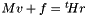n_in number of contact points mu_in array of size n giving the friction coeffs E_in array of size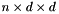giving the n normals followed by the n tangent vectors (and by again n tangent vectors if d is 3). Said otherwise, E is a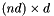matrix, stored column-major, formed by n blocks of size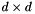with each block being an orthogonal matrix (the transition matrix from the world space coordinatesto the local coordinates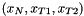w_in array of size nd, the constant term in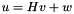ObjA array of size n, the first object involved in the i-th contact (must be an internal object) (counted from 0) ObjB array of size n, the second object involved in the i-th contact (-1 for an external object) (counted from 0) HA array of size n, containing pointers to a dense, colum-major matrix of size  d*ndof[ObjA[i]]  corresponding to the H-matrix of  ObjA[i]  HB array of size n, containing pointers to a dense, colum-major matrix of size  d*ndof[ObjA[i]]  corresponding to the H-matrix of  ObjB[i]  (NULL for an external object)
 double bogus::MecheFrictionProblem::solve ( double * r, double * v, bool staticProblem, double problemRegularization, const Options & options )

Solves the friction problem.

Parameters
 r length nd : initialization for r (in world space coordinates) + used to return computed r v length m: to return computed v ( or NULL if not needed ) staticProblem If true, do not use DeSaxce change of variable problemRegularization Amount to add on the diagonal of the Delassus operator options Solver options
 double bogus::MecheFrictionProblem::solve ( double * r, double * v, int maxThreads = 0, double tol = 0., unsigned maxIters = 0, bool staticProblem = false, double regularization = 0., bool useInfinityNorm = false, bool useProjectedGradient = false, unsigned cadouxIters = 0 )

Solves the friction problem (old interface)

Parameters
 r length nd : initialization for r (in world space coordinates) + used to return computed r v length m: to return computed v ( or NULL if not needed ) maxThreads Maximum number of threads that the GS will use. If 0, use OpenMP default. If > 1, enable coloring to ensure deterministicity tol Gauss-Seidel tolerance. 0. means GS's default maxIters Max number of iterations. 0 means GS's default staticProblem If true, do not use DeSaxce change of variable regularization Coefficient to add to the diagonal of static problems / GS regularization coefficient for friction problems useInfinityNorm Whether to use the infinity norm to evaluate the residual of the friction problem, useProjectedGradient 0 = GS, 1 = PG, 2 = ADMM, 3 = DualAMA. cadouxIters If staticProblem is false and cadouxIters is greater than zero, use the Cadoux algorithm to solve the friction problem.

The documentation for this class was generated from the following files: